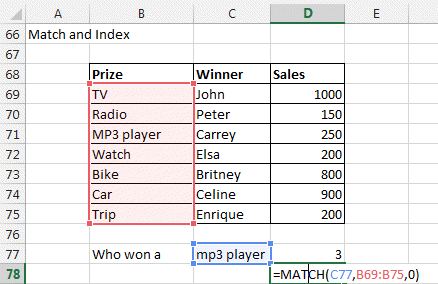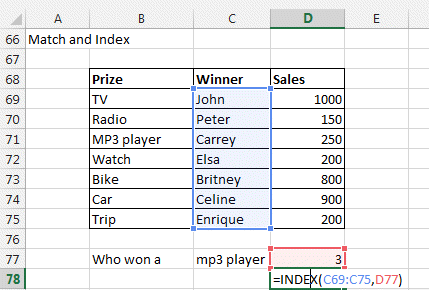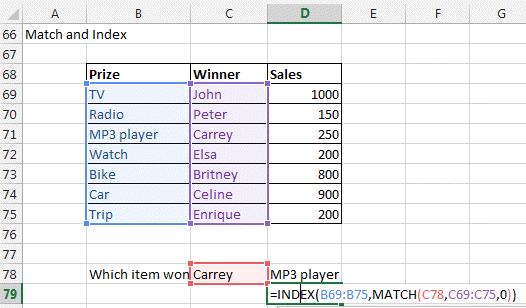## MATCH, INDEX functions in Excel

The Excel lookup functions are used to create formulas to find the specific information you search in a table.

Have a look a nice example how to use the INDEX function to select randomnly values in a table.

### MATCH

MATCH function gives you the rank in a list of items of the item you are looking for.

=MATCH(what you want to lookup, lookup column, parameter)

Parameter:

0:  if you want an exact match  (most used)

1: if you want the biggest value that is just lest or equal (list in ascending order)

-1: if you want the smalles value that is bigger or equal (list in descending order)

=Match("TV", List of items, 0)### INDEX

=INDEX(Range, row r, column c)

The INDEX function returns the value in row r and column c.

If you have only a list with 1 column, you can omit the column.

=INDEX(list of values, row r)

Have a look a nice example how to use the INDEX function to select randomnly values in a table.

Here the 3rd winner is Carrey.

=INDEX(C69:C75, 3)    gives you Carrey### Perfect LOOKUP function by COMBINING MATCH and INDEX.

By combining both functions you get a perfect search function without the limitations of VLookup or Hlookup function.

You can search in any column or row and in any order.

In our case=INDEX(prize, MATCH(C78, winners, 0) )

=INDEX(list of values, MATCH(what you want to lookup, lookup column, 0))

Hope this helped.